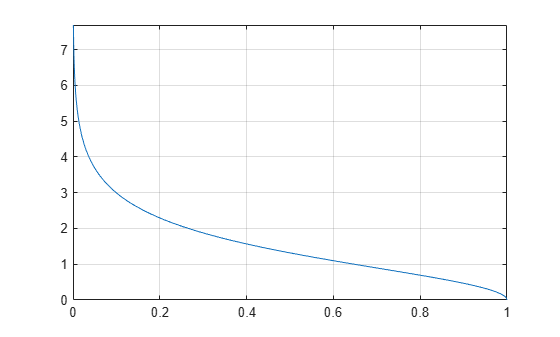# asech

Symbolic inverse hyperbolic secant function

## Syntax

``asech(X)``

## Description

example

````asech(X)` returns the inverse hyperbolic secant function of `X`.```

## Examples

### Inverse Hyperbolic Secant Function for Numeric and Symbolic Arguments

Depending on its arguments, `asech` returns floating-point or exact symbolic results.

Compute the inverse hyperbolic secant function for these numbers. Because these numbers are not symbolic objects, `asech` returns floating-point results.

`A = asech([-2, 0, 2/sqrt(3), 1/2, 1, 3])`
```A = 0.0000 + 2.0944i Inf + 0.0000i 0.0000 + 0.5236i... 1.3170 + 0.0000i 0.0000 + 0.0000i 0.0000 + 1.2310i```

Compute the inverse hyperbolic secant function for the numbers converted to symbolic objects. For many symbolic (exact) numbers, `asech` returns unresolved symbolic calls.

`symA = asech(sym([-2, 0, 2/sqrt(3), 1/2, 1, 3]))`
```symA = [ (pi*2i)/3, Inf, (pi*1i)/6, acosh(2), 0, acosh(1/3)]```

Use `vpa` to approximate symbolic results with floating-point numbers:

`vpa(symA)`
```ans = [ 2.0943951023931954923084289221863i,... Inf,... 0.52359877559829887307710723054658i,... 1.316957896924816708625046347308,... 0,... 1.230959417340774682134929178248i]```

### Plot Inverse Hyperbolic Secant Function

Plot the inverse hyperbolic secant function on the interval from 0 to 1.

```syms x fplot(asech(x),[0 1]) grid on```### Handle Expressions Containing Inverse Hyperbolic Secant Function

Many functions, such as `diff`, `int`, `taylor`, and `rewrite`, can handle expressions containing `asech`.

Find the first and second derivatives of the inverse hyperbolic secant function. Simplify the second derivative by using `simplify`.

```syms x diff(asech(x), x) simplify(diff(asech(x), x, x))```
```ans = -1/(x^2*(1/x - 1)^(1/2)*(1/x + 1)^(1/2)) ans = -(2*x^2 - 1)/(x^5*(1/x - 1)^(3/2)*(1/x + 1)^(3/2))```

Find the indefinite integral of the inverse hyperbolic secant function:

`int(asech(x), x)`
```ans = atan(1/((1/x - 1)^(1/2)*(1/x + 1)^(1/2))) + x*acosh(1/x)```

Find the Taylor series expansion of `asech(x)` around ```x = Inf```:

`taylor(asech(x), x, Inf)`
```ans = (pi*1i)/2 - 1i/x - 1i/(6*x^3) - 3i/(40*x^5)```

Rewrite the inverse hyperbolic secant function in terms of the natural logarithm:

`rewrite(asech(x), 'log')`
```ans = log((1/x - 1)^(1/2)*(1/x + 1)^(1/2) + 1/x)```

## Input Arguments

collapse all

Input, specified as a symbolic number, variable, expression, or function, or as a vector or matrix of symbolic numbers, variables, expressions, or functions.